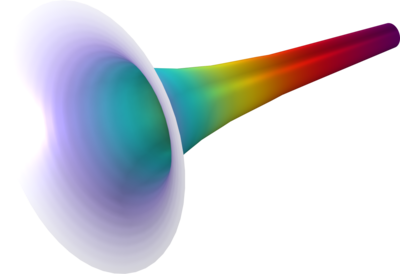# Application Gallery

## Electric Field Between Concentric Cylinders

Application ID: 105501

This introduction model creates a simple model of the electrostatics problem with two concentric cylinders of infinite length, which is commonly found in textbooks. Since there is an analytical solution to this problem, the model can be used to compare theory with numerical results from the simulation. Two cases are considered here, one with fixed potentials on both cylinders, and the other with a surface charge density on one cylinder and a fixed potential on the other.This model example illustrates applications of this type that would nominally be built using the following products: